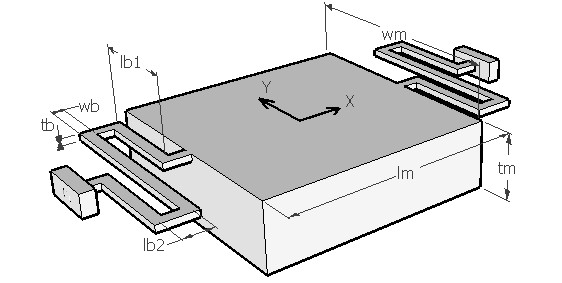SRPFRQ,lb1,lb2,wb,tb,lm,wm,tm,ns,ymod,pois,sel
Vibration frequency of a serpentine beam suspension

lb1         length of long arm of beam in µm
lb2         length of short arm of beam in µm
wb         width of the beam in µm
tb           thickness of the beam in µm
lm          length of the mass in µm
wm        width of the mass in µm
tm          thickness of the mass in µm
ns          number of serially connected serpentine beam units at each end of the suspension
ymod     Young's modulus in GPa
pois       Poisson's ratio
sel         number denoting the selected result.
Use 1 for resonant frequency in X axis, 2 for resonant frequency in Z axis and 3 for resonant frequency in torsional mode about X axisNotes

The serpentine beam is composed of two short beam segments folded around in the shape of a 'S' and connected to either end of the mass. The springs alternately compress and elongate as the mass moves along the X axis. The figure shows two such beams attached to the mass. Each of them can be formed of one or several such units connected in series, but the suspension shown in the figure has only one unit. The beams are assumed to be identical in design. This is designed to enable a translatory motion of the suspension in the X axis or in an in-plane axis. There is however a movement in the Z axis perpendicular to the plane of the mass. The mass may have tendency to rotate about the X axis also.

This design interface can be used to determine the natural vibration frequency of the folded beam suspension along the three axes. The torsional mode resonance frequency about the X axis can also be estimated. The order of resonance along these three axes will be determined by the beam and mass design.

The plot shows how the resonant frequency in X, Y and Z axes vary with the length of the smaller arm (lb2) of the serpentine beam while all other design parameters are as given in the design form. The X axis of the plot is lb2 as a percentage of lb1. Using the cross hair the resonance frequency in any of the three axes can be found out. It can be used to design the beam such that the resonant frequency for a particular axis is lesser or greater than the other axis.

Assumptions

-The default material is Silicon with density of 2330 kg/m3.
-The beam has uniform rectangular cross section.
-The mass is supported from two identical serpentine beams. The number of serial beam units are given by parameter 'ns'.
-The mass has uniform distribution of weight.
-For determining stiffness of the beam only bending effects are considered.